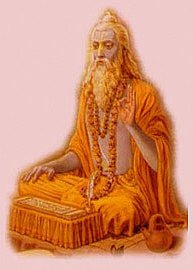# BaudhayanaBaudhayana, (fl. c. 800 BCE) was an Indian mathematician, who was most likely also a priest. He is noted as the author of the earliest Sulba Sutra—appendices to the Vedas giving rules for the construction of altars—called the Baudhayana Sulbasûtra, which contained several important mathematical results. He is older than other famous mathematician Apastambha. He belongs to Yajurveda school. He is accredited with calculating the value of pi to some degree of precision, and with DISCOVERING what is now known as the Pythagorean theorem.

### The mathematics in Shulbasutra

Pythagorean theorem

The most notable of the rules (the Sulbasutras do not contain any proofs of the rules which they describe) in the Baudhayana Sulba Sutra says:“dirghasyaksanaya rajjuH parsvamani, tiryaDaM mani, cha yatprthagbhUte kurutastadubhayan karoti.”

A rope stretched along the length of the diagonal produces an area which the vertical and horizontal sides make together. This appears to be referring to a rectangle, although some interpretations consider this to refer to a square. In either case, it states that the square of the hypotenuse equals the sum of the squares of the sides. If restricted to right-angled isosceles triangles, however, it would constitute a less general claim, but the text seems to be quite open to unequal sides.
If this refers to a rectangle, it is the earliest recorded statement of the Pythagorean theorem. Baudhayana also provides a non-axiomatic demonstration using a rope measure of the reduced form of the Pythagorean theorem for an isosceles right triangle: The cord which is stretched across a square produces an area double the size of the original square.

Circling the Square

Another problem tackled by Baudhayana is that of finding a circle whose area is the same as that of a square (the reverse of squaring the circle). His sutra i.58 gives this construction:“Draw half its diagonal about the centre towards the East-West line; then describe a circle together with a third part of that which lies outside the square.”

Explanation:

* Draw the half-diagonal of the square, which is larger than the half-side by x = {a \over 2}\sqrt{2}- {a \over 2}.

* Then draw a circle with radius {a \over 2} + {x \over 3}, or {a \over 2} + {a \over 6}(\sqrt{2}-1), which equals {a \over 6}(2 + \sqrt{2}).

* Now (2+\sqrt{2})^2 \approx 11.66 \approx {36.6\over \pi}, so the area {\pi}r^2 \approx \pi \times {a^2 \over 6^2} \times {36.6\over \pi} \approx a^2.

Square root of 2

Baudhayana i.61-2 (elaborated in Apastamba Sulbasutra i.6) gives the length of the diagonal of a square in terms of its sides, which is equivalent to a formula for the square root of 2:samasya dvikarani. pramanam trtiyena vardhayet tac caturthenatmacatustrimsonena savisesah

The diagonal of a square. The measure is to be increased by a third and by a fourth decreased by the thirty-fourth. That is its diagonal approximately. \sqrt{2} = 1 + \frac{1}{3} + \frac{1}{3 \cdot 4} – \frac{1}{3 \cdot4 \cdot 34} = \frac{577}{408} \approx 1.414216 which is correct to five decimals. Other theorems include: diagonals of rectangle bisect each other, diagonals of rhombus bisect at right angles, area of a square formed by joining the middle points of a square is half of original, the midpoints of a rectangle joined forms a rhombus whose area is half the rectangle, etc. Note the emphasis on rectangles and squares; this arises from the need to specify yajña bhumikas—i.e. the altar on which a rituals were conducted, including fire offerings (yajña). Apastamba (c. 600 BC) and Katyayana (c. 200 BC), authors of other sulba sutras, extend some of Baudhayana’s ideas. Apastamba provides a more general proof[citation needed] of the Pythagorean theorem.